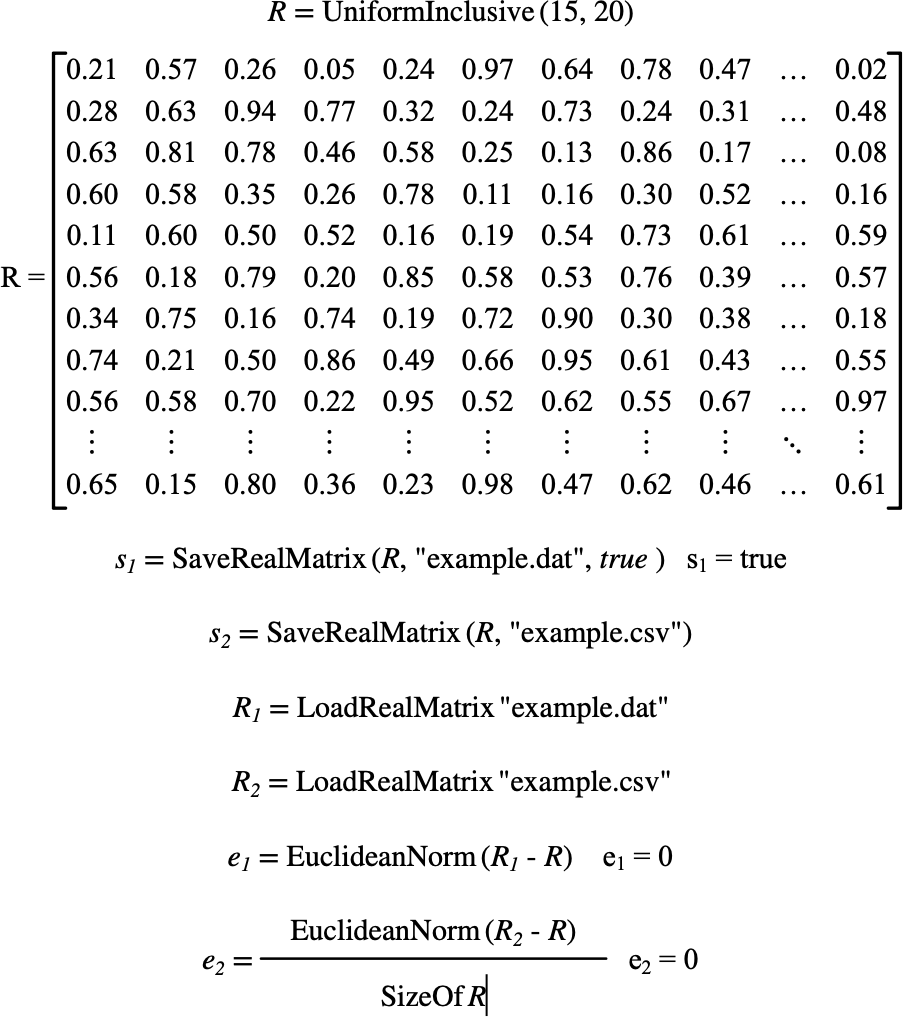# $$\text{LoadRealMatrix}$$¶

You can use the $$\text{LoadRealMatrix}$$ to load a real matrix from a file.

You can use the \loadrealmatrix backslash command to insert this function.

The following variants of this function are available:

• $$\text{real matrix } \text{LoadRealMatrix} \left ( \text{<filename>} \right )$$

The $$\text{<filename>}$$ parameter is the filename of the file to be loaded.

The function returns the newly loaded real matrix. The matrix can be stored either in CSV format or in a simple packed binary format. The file type is determined by checking for a file magic number stored at the beginning of the file.

The CSV file can use most common separator characters to separate column values with column values written as pairs of real/imaginary values. Supported column separators include tabs, spaces, commas (,), semicolons (;), vertical bars (|), or colons (:). The matrix size is determined based on the maximum number of entries per line and the number of lines.

The binary format is designed to be relatively simple for external applications to implement. For details on the binary format, see File Formats.

The Figure 170 shows how you can use the $$\text{LoadRealMatrix}$$ function.Figure 170 Example Use Of The LoadRealMatrix Function